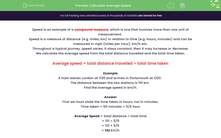# Calculate Average Speed

In this worksheet, students will calculate average speeds given, total distance travelled and total time taken to complete a journey.Key stage:  KS 3

Curriculum topic:   Geometry and Measures

Curriculum subtopic:   Derive and Apply Formulae

Difficulty level:#### Worksheet Overview

Speed is an example of a compound measure, which is one that involves more than one unit of measurement.

Speed is a measure of distance (e.g. miles, km) in relation to time (e.g. hours, minutes) and can be measured in mph (miles per hour), km/h etc.

Throughout a typical journey, speed varies. It stays constant, then it may increase or decrease.

We calculate the average speed from the total distance travelled and the total time taken.

Average speed = total distance travelled ÷ total time taken

Example

A train leaves London at 11:30 and arrives in Portsmouth at 12:20.

The distance between the two stations is 110 km.

Find the average speed in km/h.First, we must state the time taken in hours, not in minutes.

Time taken = 50 minutes = 50/60 which is simplified to 5/6 hour.

Average Speed = total distance ÷ total time

= 110 ÷ 5/6

= 110 × 6/5

= 132 km/h

The average speed is 132 km/h

To test and consolidate your knowledge we'll use it to answer word problems!

### What is EdPlace?

We're your National Curriculum aligned online education content provider helping each child succeed in English, maths and science from year 1 to GCSE. With an EdPlace account you’ll be able to track and measure progress, helping each child achieve their best. We build confidence and attainment by personalising each child’s learning at a level that suits them.

Get started••••